## Reflection Property

In the plane, the reflection property can be stated as three theorems (Ogilvy 1990, pp. 73-77):

1. The Locus of the center of a variable Circle, tangent to a fixed Circle and passing through a fixed point inside that Circle, is an Ellipse.

2. If a variable Circle is tangent to a fixed Circle and also passes through a fixed point outside the Circle, then the Locus of its moving center is a Hyperbola.

3. If a variable Circle is tangent to a fixed straight line and also passes through a fixed point not on the line, then the Locus of its moving center is a Parabola.

Let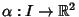be a smooth regular parameterized curve in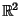defined on an Open Interval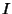, and let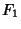and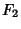be points in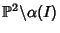, where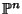is an-D Projective Space. Then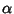has a reflection property with Fociandif, for each point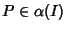,

1. Any vector normal to the curveat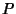lies in the Span of the vectors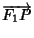and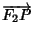.

2. The line normal toatbisects one of the pairs of opposite Angles formed by the intersection of the lines joiningandto.
A smooth connected plane curve has a reflection property Iff it is part of an Ellipse, Hyperbola, Parabola, Circle, or straight Line.

 Foci Sign Both foci finite One focus finite Both foci infinite distinct Positive confocal ellipses confocal parabolas parallel lines distinct Negative confocal hyperbola and perpendicular confocal parabolas parallel lines bisector of interfoci line segment equal concentric circles parallel lines

Let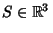be a smooth connected surface, and letandbe points in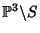, whereis an-D Projective Space. Then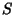has a reflection property with Fociandif, for each point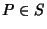,

1. Any vector normal toatlies in the Span of the vectorsand.

2. The line normal toatbisects one of the pairs of opposite angles formed by the intersection of the lines joiningandto.
A smooth connected surface has a reflection property Iff it is part of an Ellipsoid of revolution, a Hyperboloid of revolution, a Paraboloid of revolution, a Sphere, or a Plane.

 Foci Sign Both foci finite One focus finite Both foci infinite distinct Positive confocal ellipsoids confocal paraboloids parallel planes distinct Negative confocal hyperboloids and plane perpendicular confocal paraboloids parallel planes bisector of interfoci line segment equal concentric spheres parallel planes

References

Drucker, D. Euclidean Hypersurfaces with Reflective Properties.'' Geometrica Dedicata 33, 325-329, 1990.

Drucker, D. Reflective Euclidean Hypersurfaces.'' Geometrica Dedicata 39, 361-362, 1991.

Drucker, D. Reflection Properties of Curves and Surfaces.'' Math. Mag. 65, 147-157, 1992.

Drucker, D. and Locke, P. A Natural Classification of Curves and Surfaces with Reflection Properties.'' Math. Mag. 69, 249-256, 1996.

Ogilvy, C. S. Excursions in Geometry. New York: Dover, pp. 73-77, 1990.

Wegner, B. Comment on `Euclidean Hypersurfaces with Reflective Properties'.'' Geometrica Dedicata 39, 357-359, 1991.